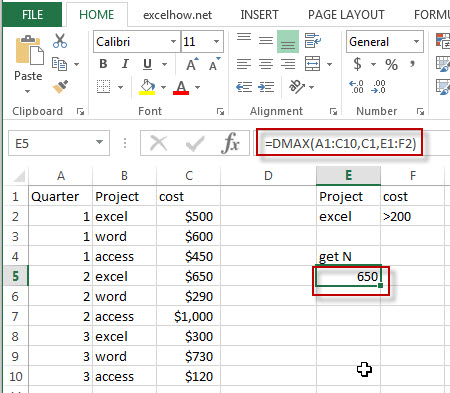# Excel Dmax Function

This post will guide you how to use Excel DMAX function with syntax and examples in Microsoft excel.

### Description

The Excel DMAX returns the maximum value from a database or a column of a list that matches the specified conditions.

The DMAX function is a build-in function in Microsoft Excel and it is categorized as a Database Function.

The DMAX function is available in Excel 2016, Excel 2013, Excel 2010, Excel 2007, Excel 2003, Excel XP, Excel 2000, Excel 2011 for Mac.

### Syntax

The syntax of the DMAX function is as below:

`= DMAX (database, field, criteria)`

Where the DMAX function arguments are:

• Database -This is a required argument.  The range of cells that containing the database.
• Field – This is a required argument.  The column with database that you want the maximum of.
• Criteria – The range of cells that contains the conditions that you specify.

### Excel DMAX Function Examples

The below examples will show you how to use Excel DMAX Function to find the maximum value from a column of a list or database that matches a given criteria.

#1 = DMAX (A1:C10,C1,E1:F2)Note: The above excel formula will find excel project and its cost is greater than 200, then return the maximum one(650).

Related Functions

• Excel DMIN Function
The Excel DMIN returns the minimum value from a database or a column of a list that matches the specified conditions.The syntax of the DMIN function is as below:=DMIN(database, field, criteria)…